•研究论文
•研究论文
•研究论文
• Gauss投影的复变函数表示.利用复变函数理论重新讨论了高斯投影.
•研究论文
•研究论文
• D3DXMatrixShadow 产生一个矩阵，把几何体投影到平面上，神奇是这个矩阵不论对平行光还是点光，都具有统一形式。 随着点光源距离平面越来越远，平面上任意两点接收到光线趋于平行，若点光源位置无穷远，则就是...
D3DXMatrixShadow 产生一个矩阵，把几何体投影到平面上，神奇的是这个矩阵不论对平行光还是点光，都具有统一的形式（见左边D3DXMatrixShadow的文档链接）。
Introduction to 3D Game Programming with DirectX9.0 模板缓冲区那一章里提示感兴趣的读者参考Chapter 6, “Me and My (Fake) Shadow,” Jim Blinn’s Corner: A Trip Down the Graphics Pipeline。
《计算机图形学与几何造型导论》用很基本的向量和矩阵（线性代数）的知识，分别推导出了平行投影和透视投影各自的变换矩阵。因为后者我很早就读过了，非常熟悉；只把前者简单地看了看，有了一个思路（随着点光源距离平面越来越远，平面上任意两点接收到的光线趋于平行，若点光源位置无穷远，则就是平行光了），把这两个变换矩阵合二为一。

齐次坐标
一维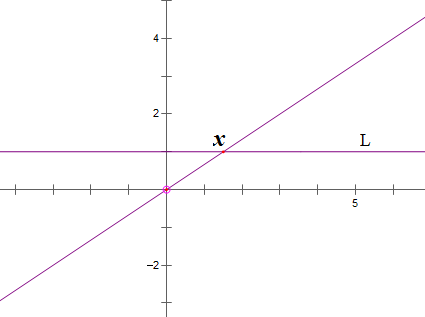数轴L上一点的坐标 x = x/1 = kx/k ( k!=0 ) 即数轴上一点的坐标可以写成两个实数 X、k 的比值，记作 (X,k) k!=0，这就是一维空间点的齐次坐标（比例关系X:k）。
(x,1) 与 k!=0 k(x,1)=(kx,k) 都是数轴L上的同一个点，对应着二维空间中的一条过原点、斜率不为零的直线（除去原点）。
(X,k) k!=0 代表数轴L上的点 x=X/k，若X!=0，k->0  x-> infinity，即无穷远。记 (X,0) X!=0 代表无穷远，对应着二维空间中过原点、斜率为零的直线（除去原点）。
而剩下的 (0,0) 对应着 二维空间的 原点。

二维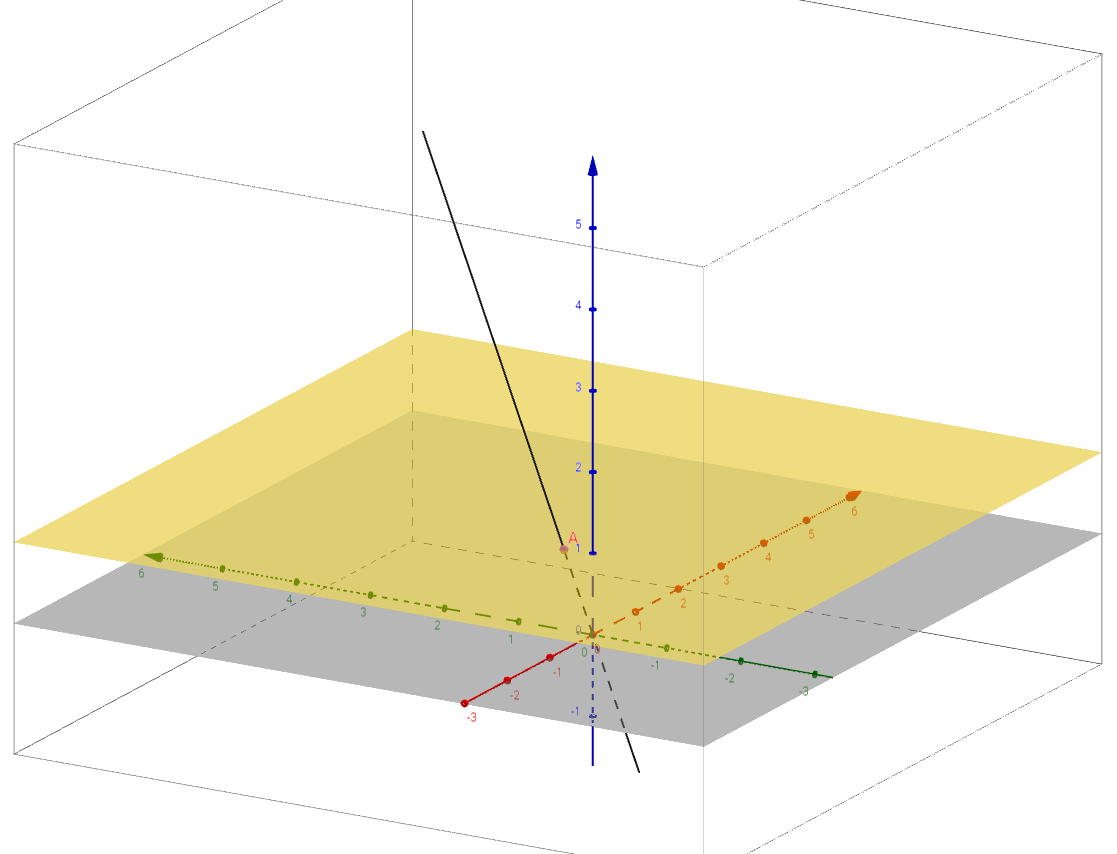黄色平面（z=1）上某点A的二维坐标 (x,y) = (x/1, y/1) = (kx/k, ky/k) k!=0，kx=X，ky=Y，写成齐次坐标的形式：(X, Y, k) <--> (X/k, Y/k, 1) = (x,y,1) k!=0，比例关系 (X: Y :k) 。
(x,y,1) 与 (kx,ky,k) k!=0 都表示黄色平面上的同一个点，对应着三维空间中一条过原点、不在xoy平面（灰色）内的直线（除去原点）。
(X, Y, k) k!=0 代表黄色平面上的点(x,y) x=X/k, y=Y/k，k->0, 若X,Y不同时为零，(x,y)= (X,Y)*(1/k) 沿(X,Y)方向趋于无穷远。记 (X,Y,0)代表无穷远，对应着三维空间中过原点、在xoy平面上、方向向量是(X,Y)的直线（除去原点）。
而剩下的 (0,0,0) 对应着 三维空间的 原点。
在黄色平面这个二维世界中，A是一个点光源，它的齐次坐标为 (a,b,w)，即二维坐标为 (a/w,b/w) = (a,b)*(1/w)，当w->0时，点A不断沿着平行于向量(a,b)的方向（与向量(a,b)的方向相同或相反）向远处移动，最后趋于黄色平面上向量(a,b)方向的直线两端的无穷远。此时A就是一个平行光了，方向是(a,b)。记作(a,b,0)。(a,b,0) 与 (ka,kb,0) k!=0 产生的平行光线是一样的。
这样平行光、点光源就用齐次坐标统一起来了：(a,b,w) w!=0 时，代表(a/w,b/w)位置的点光源；w=0且a、b不同时为零，代表平行于向量(a,b)的平行光。(0,0,0)含义未定义。
无穷远 对应 一组共线的非零向量。

同理可以推广到三维空间、更高维度几何空间点的齐次坐标，运用代数。

投影矩阵
平行投影
投影平面过Q点，法向是N，平行光线的方向向量为u（光线与平面不平行），则投影矩阵
Parallel(Q,N,u) 为（证明见附录），矩阵的值与被变换的点P(x,y,z,1)无关：
$$\begin{bmatrix} I-\frac{N^\top u}{uN^\top} & 0 \\ \\ \frac{QN^\top}{uN^\top}u & 1 \\ \end{bmatrix}$$

透视投影
投影平面过Q点，法向是N，点光源的位置为u，则投影矩阵 Perspective(Q,N,u) 为（证明见附录），矩阵的值与被变换的点P(x,y,z,1)无关：

$$\begin{bmatrix} (u-Q)N^\top I-N^\top u & -N^\top \\ \\ QN^\top u & uN^\top \\ \end{bmatrix}$$
齐次形式
(x,y,z,1) Parallel(Q,N,u) = (x',y',z',1)
(x,y,z,1) Parallel(Q,N,u)uN^T = (x',y',z',1) uN^T ~ (x',y',z';1)
$$Parallel(Q,N,u)uN^\top=\begin{bmatrix}uN^\top I-N^\top u & 0 \\ \\QN^\top u & uN^\top \\\end{bmatrix}$$
> Parallel(Q,N,u)uN^T也是平面Q,N和平行光u的平行投影矩阵。

点光源的位置是u，若表示成齐次坐标(S,w)，w是任意不为零的常数，则u=S/w，代入Perspective(Q,N,u)得：
$$Perspective(Q,N,S,w)=\begin{bmatrix} (\frac{1}{w}S-Q)N^\top I-\frac{1}{w}N^\top S & -N^\top \\ \\ \frac{1}{w}QN^\top S & \frac{1}{w}SN^\top \\ \end{bmatrix}\quad w\neq 0$$
(x,y,z,1) Perspective(Q,N,S,w) = (X,Y,Z,k)  ~ (X/k,Y/k,Z/k,1)
(x,y,z,1) Perspective(Q,N,S,w) w =  (X,Y,Z,k) w ~ (X/k,Y/k,Z/k,1)w!=0
$$Perspective(Q,N,S,w)w=\begin{bmatrix} (S-wQ)N^\top I-N^\top S & -wN^\top \\ \\ QN^\top S & SN^\top \\ \end{bmatrix}\quad w\neq 0$$
> w!=0，Perspective(Q,N,S,w) w 是平面Q,N和点光源(S,w)的透视投影矩阵。
统一的表示
L=(a,b,c,w)=(S,w), a,b,c,w 不同时为零，定义一个矩阵叫做Projection(L,Q,N) 为：$$\begin{bmatrix} (S-wQ)N^\top I-N^\top S & -wN^\top \\ \\ QN^\top S & SN^\top \\ \end{bmatrix}$$形式上与Perspective(Q,N,S,w) w完全一样。

w=!0时，L是点光源，其透视投影矩阵是Perspective(Q,N,S,w) w，也就是Projection(L,Q,N)。
现在对于任意的一个给定的S，让w趋向于0，点光源的位置沿着S方向的直线移动；极限情况下 L是一个与S共线的平行光，lim L = lim (S,w) = (S,0)；
而w->0极限情况下 lim Projection(L,Q,N)= Parallel(Q,N,S)SN^T，是一个平行投影矩阵。（求极限都是把w=0带入）

所以，Projection(L,Q,N)在w!=0时表示点光源的透视投影矩阵，在w=0时表示平行光的平行投影矩阵。

附录
平行投影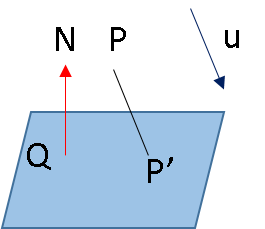字母既代表 点或（行）向量，也代表它们的坐标，运用向量代数：
$$\begin{array}{ll} P' = P+ tu \\ (P'-Q)N^\top = 0\end{array}$$
解得 $$t = {{QN^\top-PN^\top} \over {uN^\top}}$$,
所以 $$P' = P+ \frac{QN^\top u-PN^\top u}{uN^\top} = P + \frac{QN^\top u}{uN^\top} - \frac{PN^\top u}{uN^\top} =P (I-\frac{N^\top u}{uN^\top}) + \frac{QN^\top}{uN^\top}u$$.
$$v' = P'-Q = P (I-\frac{N^\top u}{uN^\top}) + Q(\frac{N^\top u}{uN^\top}-I) = (P-Q) (I-\frac{N^\top u}{uN^\top})= v (I-\frac{N^\top u}{uN^\top})$$.
符合仿射变换的定义：P'=PM+w，v'=vM，得：
$$M=(I-{N^\top u \over uN^\top}),w={QN^\top \over uN^\top} u$$
透视投影
质点几何
①把位置是P，质量是m!=0的点记做(mP,m)，叫做质点坐标。已知某点的质点坐标很容易求出它的位置：mP/m=P。
(mP,m)~(P,1)，~ 代表等价关系（自反、对称、传递的二元关系），a~b: a b同一位置。
②质点坐标的好处是质心位置的计算：A(mP,m)，B(nQ,n)，则A、B的质心为(mP+nQ, m+n) ~ ( (mP+nQ)/(m+n), 1)。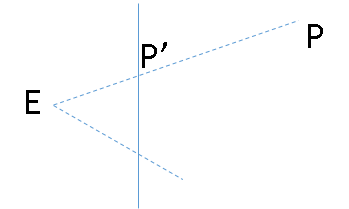E是投影点，P' 位于投影平面上，该平面过点Q，法向是N（单位法向量），假定方向朝右（朝左不影响的）
E到平面的距离=d，P到平面的距离=z，
假设点E的质量=z，点P的质量=d，
则P' 所在的位置恰好是E、P两点的质心（杠杆EP的支点）。

透视投影矩阵
(E,1)~(Ez,z)，(P,1)~(Pd,d)，
(Ez,z)+(Pd,d) = (Ez+Pd, z+d) ~ (P',1)

其中，$$z=(P-Q)N^\top, \;d=(Q-E)N^\top, \;z+d=(P-E)N^\top$$

所以，$$(Ez+Pd, z+d)=( (P-Q)N^\top E+(Q-E)N^\top P, \; (P-E)N^\top )$$

$$=( PN^\top E -QN^\top E +(Q-E)N^\top P, \; PN^\top -EN^\top)$$

$$=( P(N^\top E+(Q-E)N^\top I) -QN^\top E, \; PN^\top -EN^\top)$$

假设$$(P,1)\begin{pmatrix} M & b \\ w & c \\ \end{pmatrix}_{H} =(Ez+Pd, z+d) \sim (P',1)$$

则$$M=N^\top E+(Q-E)N^\top I, \; w=-QN^\top E, \; b= N^\top, c=-EN^\top$$

因为 k (Ez+Pd, z+d)=( k(Ez+Pd), k(z+d) ) ~ (Ez+Pd, z+d) ~ (P',1) k!=0
所以 矩阵H可以 相差非零因子倍，于是法向朝左或朝右没有关系。


展开全文•研究论文
• crp matlab代码CRP_Repro 用于协作表示投影的 Matlab 代码
• 使用投影矩阵和恢复矩阵近似地表示隐式低秩表示的投影矩阵,使算法在降维同时可提取判别特征;联合学习投影矩阵、恢复矩阵和低秩矩阵,矩阵间相互提升,从获得的投影中可以提取出更多判别特征,同时在算法模型中...
• 借助复变函数理论讨论了常用等角投影及其解析变换复变函数表示；给出了高斯投影、墨卡托投影和等角圆锥投影正反解复变函数表示模型；在此基础上系统地推导出了高斯投影、墨卡托投影和等角圆锥投影间解析变换复...
• 文中论述了二维平面成像的投影变换模型并给出其单应矩阵表示 详细推导。
• 基于稀疏表示分类鉴别投影（SRC steered discriminative projection，SRC-DP）则是建立在SRC分类准则基础上降维方法，其在投影空间中最大化类间重构误差与类内重构误差比值。针对SRC-DP中提取特征之间具有...特征提取
• 本文讨论了最佳线性预测在Hilbert空间中表现形式，并引出了Wold表示定理。
文章目录十七、Hilbert空间的投影与Wold表示定理1.Hilbert空间上的投影2.线性闭包与无穷历史预测3.Wold表示定理回顾总结
十七、Hilbert空间的投影与Wold表示定理
1.Hilbert空间上的投影
在《四、Hilbert空间》中，我们提到了随机变量的Hilbert空间，这是一个二阶矩有限的随机变量全体构成的线性空间，并且是一个内积空间、度量空间、完备空间（完备性指的是基本列总存在极限）。在介绍了最佳线性预测的概念后，我们也可以在Hilbert空间上找到最佳线性预测的几何意义。
$Y$关于$\boldsymbol X$的最佳线性预测，是用$\boldsymbol X$分量的线性组合表示$Y$的所有与$\boldsymbol X$相关的部分，这意味着$Y-L(Y|\boldsymbol X)$与$\boldsymbol X$不相关。而在Hilbert空间中，对内积的定义是$\langle X,Y\rangle={\rm E}(XY)$，所以$X,Y$正交实际上指的就是$X,Y$不相关。于是，我们可以认为$L(Y|\boldsymbol X)$是$Y$在$\boldsymbol X$上的投影。
在证明这个结论之前，先对投影与垂直（随机变量与空间）作出定义。

投影：如果$H$是$L^2$的闭子空间，$Y\in L^2,\hat Y\in H$使得
${\rm E}(Y-\hat Y)^2=\inf_{\xi\in H}{\rm E}(Y-\xi)^2,$
就称$\hat Y$是$Y$在$H$上的投影，记作$\hat Y=P_H(Y)$，并且称$P_H$为投影算子。
垂直：设$Y\in L^2$，如果$H$中的任何$\xi$都有${\rm E}(Y\xi)=0$，就称$Y$垂直于$H$。
投影与垂直的关系：设$Y\in L^2,\hat Y\in H$，则
$P_H(Y)=\hat Y\Leftrightarrow (Y-\hat Y)\perp H.$

投影的存在性不再证明，只需要知道对于任何$L^2$的闭子空间$H$，在$L^2$内的随机变量$Y$都能在$H$内找到唯一的投影$\hat Y=P_H(Y)$即可。
这样，我们就能够通过${\rm E}[\boldsymbol X(Y-L(Y|\boldsymbol X))]=0$得到垂直，从而证明了$L(Y|\boldsymbol X)$是$Y$在$\text{sp}(\boldsymbol X)$上的投影，因此，我们还可以把投影算子$P_H(Y)$记作$L(Y|H)$。从最佳线性预测的性质出发，还能够得到以下关于最佳线性预测与投影的性质：

$L(aX+bY|H)=aL(X|H)+bL(Y|H)$——投影的线性性。

${\rm E}Y^2={\rm E}[L(Y|H)]^2+{\rm E}[Y-L(Y|H)]^2$——Hilbert空间上的勾股定理。

${\rm E}[L(Y|H)]^2\le {\rm E}Y^2$——直角三角形中的不等式。

$Y\in H\Leftrightarrow L(Y|H)=Y$——空间性质。

$Y\perp H\Leftrightarrow L(Y|H)=0$——空间性质。

如果$H\sub M$，则$P_HP_M=P_H$，且对$Y\in L^2$，有
${\rm E}[Y-L(Y|M)]^2\le {\rm E}[Y-L(Y|H)]^2,$
从最佳线性预测角度看，$M$包含了比$H$更多的信息；从Hilbert空间的角度看，与高维的距离总是大于与低维度的距离。

最后简要提一下Hilbert空间中的最佳预测。最佳预测与最佳线性预测的区别在于，它去除了线性的限制，这使得用来拟合目标随机变量的式子形式更多样，也因此更能缩小误差。在最佳线性预测中，我们采用$\boldsymbol X$的线性组合构成的Hilbert空间$H$，进入最佳预测时，我们考虑的预测空间就变成了$\boldsymbol X$的所有二阶矩有限函数构成的空间，即
$M=\{g(\boldsymbol X):{\rm E}g^2(\boldsymbol X)<\infty \}.$
最佳预测自然应该满足$L(Y|M)\perp M$，而显然有$H\sub M$，所以最佳预测的均方误差也不超过最佳线性预测。但由于最佳预测的形式复杂（在概率论中说过，最佳预测就是条件期望），所以在使用上没有最佳线性预测方便，并且在正态条件下，最佳线性预测就是最佳预测，这是因为正态分布的不相关与等价独立。

最佳线性预测就是最佳预测的条件：设$(X_1,\cdots,X_n,Y)'=(\boldsymbol X',Y)'$服从联合正态分布$N_{n+1}(\boldsymbol \mu,\Sigma)$，则
$L(Y|M)=L(Y|\boldsymbol X).$

2.线性闭包与无穷历史预测
最佳线性预测中，我们用到的是$\boldsymbol X$的线性组合构成的空间，记作${\rm sp}(\boldsymbol X)$，但这里$\boldsymbol X$是有限维的，如果我们要将$\boldsymbol X$的维数扩展到无限维，就需要对极限情况加以更多的讨论。而Hilbert空间是完备的，因而极限不可忽视，于是引入线性闭包$\overline{\rm sp}(A)$。

线性闭包：设$A$是Hilbert空间$H$的子集，令$A$的线性闭包为包含$A$的最小的闭子空间$\overline{\rm sp}(A)=H_A$。这样，$H_A$是完备的，即$\forall \xi\in H_A,\exists \xi_n\in H_A$使得
$||\xi_n-\xi||\to 0(n\to \infty).$

事实上，$H_A$就是${\rm sp}(A)$中元素及其极限构成的集合，且是一个闭集。
因为我们假定平稳序列拥有无穷多的历史信息，所以有了线性闭包这一工具，我们就可以转向平稳序列的预测了。求对平稳序列的最佳线性预测，就是在给定一系列历史$X_n,X_{n-1},\cdots$后，在无穷历史的线性闭包$H_n$上求$L(X_{n+k}|H_n)$。根据$H_n$的完备性，我们可以由有穷历史逼近无穷历史，相关定理如下：

使用无穷历史进行预测：设$\boldsymbol X_{n,m}=(X_n,X_{n-1},\cdots,X_{n-m+1})'$，$Y\in L^2$，则当$m\to \infty$时，
$L(Y|\boldsymbol X_{n,m})\stackrel{\rm m.s.}\to L(Y|H_n)\stackrel {\rm d}=\hat Y.$

证明过程是构造一系列随机变量$\hat Y_m$用来逼近$\hat Y$，从而由Hilbert空间的性质得到最佳线性预测的均方相合性，自然地$\hat Y_m=L(Y|\boldsymbol X_{n,m})$。
此时，如果我们把$Y$换成$X_{n+1},\cdots,X_{n+k}$，就有
$\sigma_1^2={\rm E}[X_{n+1}-L(X_{n+1}|H_n)]^2={\rm E}[X_1-L(X_{1}|H_0)]^2,\\ \vdots \\ \sigma^2_k={\rm E}[X_{n+k}-L(X_{n+k}|H_n)]^2={\rm E}[X_{k}-L(X_k|H_0)]^2.$
结合我们对决定性平稳序列、非决定性平稳序列的定义，可以得到如下的结论：

决定性平稳序列：$\{X_t\}$是决定性平稳序列，等价于$\sigma_1^2=0$，即$X_{n+1}\in H_n$，也就有$H_n=H_{n-1}$。
纯非决定性平稳序列：$\{X_t\}$是纯非决定性平稳序列，等价于$\sigma_k^2\to \gamma_0$，也就是
$\sigma^2_k=||X_{n+k}-L(X_{n+k}|H_n)||^2\to \gamma_0\quad (k\to \infty).$

3.Wold表示定理
Wold表示定理是一种对非决定性零均值平稳序列的拆分，它将一个非决定性平稳序列拆分成不相关的纯非决定性的部分与决定性的部分，内容如下。

Wold表示定理：任一非决定性的零均值平稳序列$\{X_t\}$可以表示成
$X_t=\sum_{j=0}^\infty a_j\varepsilon_{t-j}+V_t,\quad t\in\Z,$
其中，

$\varepsilon_t=X_t-L(X_t|X_{t-1},X_{t-2},\cdots)$是零均值白噪声，满足
${\rm E}\varepsilon_t^2=\sigma^2>0,\quad a_0=1,\\ a_j=\frac{{\rm E}(X_t\varepsilon_{t-j})}{\sigma^2},\\ \sum_{j=0}^\infty a_j^2<\infty.$

定义$U_t=\sum_{j=0}^\infty a_j\varepsilon_{t-j}$，则$\{U_t\},\{V_t\}$都是平稳序列，且互相正交。

定义$H_\varepsilon(t)=\overline{\rm sp}\{\varepsilon_s:s\le t\},H_U(t)=\overline{\rm sp}\{U_s,s\le t\}$，则
$H_\varepsilon(t)=H_U(t).$

$\{U_t\}$是纯非决定性的平稳序列，谱密度为
$f(\lambda)=\frac{\sigma^2}{2\pi}\left|\sum_{j=0}^\infty a_je^{{\rm i}j\lambda} \right|^2.$

$\{V_t\}$是决定性的平稳序列，且对任何$t,k\in\Z$，$V_t\in H_{t-k}$。

对平稳序列的Wold表示，作如下的定义：

称$X_t=U_t+V_t$是$\{X_t\}$的Wold表示。
称$\{U_t\}$是$\{X_t\}$的纯非决定性部分，$\{V_t\}$是$\{X_t\}$的决定性部分。
称$\{a_j\}$是$\{X_t\}$的Wold系数。
称一步预测误差$\varepsilon_t=X_t-L(X_t|X_{t-1},\cdots)$是$\{X_t\}$的新息序列。
称$\sigma^2={\rm E}\varepsilon_t^2$为一步预测的均方误差。

可以看到，Wold表示定理把非决定序列分成的两部分中，纯非决定性的部分是白噪声的单边无穷滑动和，这与${\rm AR}(p)$序列与${\rm ARMA}(p,q)$序列的一致，并且，白噪声的系数也同样被称为Wold系数。而新息序列，就是在非决定性部分中，用历史对下一步作预测的误差，误差的方差就是一步预测的均方误差。
为了计算误差的方差，有Kolmogorov公式：

Kolmogorov公式：设$\{U_t\}$是$\{X_t\}$的纯非决定性部分，$f(\lambda)$是$\{U_t\}$的谱密度，则其一步预测误差的方差为
$\sigma^2=2\pi\exp\left(\frac1{2\pi}\int_{-\pi}^\pi\ln f(\lambda){\rm d}\lambda \right).$

有了一步预测的误差，还可以计算$n$步预测误差的方差，即$\sigma^2(n)={\rm E}[X_{t+n}-L(X_{t+n}|H_n)]^2$，因为
$X_{t+n}-L(X_{t+n}|H_n)=\sum_{j=0}^{n-1}a_j\varepsilon_{t+n-j},$
所以均方误差为
$\sigma^2(n)=\sigma^2\sum_{j=0}^{n-1}a_j^2\to {\rm E}U_t^2\quad (n\to \infty).$
回顾总结

最佳线性预测$L(Y|\boldsymbol X)$，其实就是$Y$在${\rm sp}(\boldsymbol X)$上的投影，因此$L(Y|\boldsymbol X)$具有的性质都可以在Hilbert空间上导出。

如果将$\boldsymbol X$的所有可测函数中，二阶矩有限的随机变量构成一个新空间$M$，那么$Y$在$M$空间上的投影$L(Y|M)$就是$\boldsymbol X$对$Y$的最佳预测。在正态约束下，最佳预测与最佳线性预测相等。

如果$\boldsymbol X$是无限维的，那么$\boldsymbol X$的有限线性组合及其极限构成的集合是一个闭集，称为线性闭包，记作$H_\boldsymbol X$或$\overline{\rm sp}(\boldsymbol X)$。使用无穷历史信息进行线性预测，其实就是在线性闭包上作投影，对于时间序列$\{X_n\}$来说，$n$时刻以前的历史信息构成的线性闭包又记作$H_n$。

记$\sigma^2_k={\rm E}[X_{n+k}-L(X_{n+k}|H_n)]$，则$\sigma_1^2=0$等价于$\{X_n\}$是决定性的，$\sigma_k^2\to \gamma_0$等价于$\{X_t\}$是纯非决定性的。

Wold表示定理指出，任何零均值平稳序列，都可以拆分成两个不相关的纯非决定性部分与决定性部分的加和。这里纯非决定性部分是白噪声的单边无穷滑动和，白噪声序列称为新息序列，前面的系数称为Wold系数，白噪声方差就是一步预测的均方误差。

Kolmogorov联系起了一步预测的均方误差与谱密度，存在这样的关系：
$\sigma^2=2\pi\exp\left(\frac 1{2\pi}\int_{-\pi}^\pi \ln f(\lambda){\rm d}\lambda \right).$
同时有$\sigma_k^2=\sigma^2\sum_{j=0}^k a_j^2,\sigma^2_k\to {\rm E}U_t^2$。


展开全文•matlab
• tsne词嵌入 用来投射单词的程序。 它有助于可视化单词在向量空间中的表示效率 输入：Gloves25.txt：包含名称的文本文件，其矢量表示为25维 --->通过运行python project.py运行代码Python
• 引言当面对数据被抽象为一组向量，那么有必要研究一些向量数学性质。而这些数学性质将成为PCA理论基础。理论描述向量运算即：内积。首先，定义两个维数相同向量内积为：(a1,a2,⋯,an)T⋅(b1,b2,⋯,bn)T=a1...


原文：http://blog.csdn.net/songzitea/article/details/18219237
引言

当面对的数据被抽象为一组向量，那么有必要研究一些向量的数学性质。而这些数学性质将成为PCA的理论基础。
理论描述

向量运算即：内积。首先，定义两个维数相同的向量的内积为：

(a1,a2,⋯,an)T⋅(b1,b2,⋯,bn)T=a1b1+a2b2+⋯+anbn

内积运算将两个向量映射为一个实数。其计算方式非常容易理解，但是其意义并不明显。所以，我们分析内积的几何意义。假设A和B是两个n维向量，我们知道n维向量可以等价表示为n维空间中的一条从原点发射的有向线段，为了简单起见我们假设A和B均为二维向量，则A=(x1,y1)，B=(x2,y2)。则在二维平面上A和B可以用两条发自原点的有向线段表示，如图1所示。

现在，我们从A点向B所在直线引一条垂线。我们知道垂线与B的交点叫做A在B上的投影，再设A与B的夹角是a，则投影的矢量长度为|A|cos(a)，其中|A|=x21+y21−−−−−−√是向量A的模，也就是A线段的标量长度。注意这里我们专门区分了矢量长度和标量长度，标量长度总是大于等于0，值就是线段的长度；而矢量长度可能为负，其绝对值是线段长度，而符号取决于其方向与标准方向相同或相反。到这里还是看不出内积和这东西有什么关系，不过如果我们将内积表示为另一种我们熟悉的形式：

A⋅B=|A||B|cos(a)

现在事情似乎是有点眉目了：A与B的内积等于A到B的投影长度乘以B的模。再进一步，如果我们假设B的模为1，即让|B|=1，那么就变成了：

A⋅B=|A|cos(a)

也就是说，设向量B的模为1，则A与B的内积值等于A向B所在直线投影的矢量长度！这就是内积的一种几何解释，也是我们得到的第一个重要结论。在后面的推导中，将反复使用这个结论。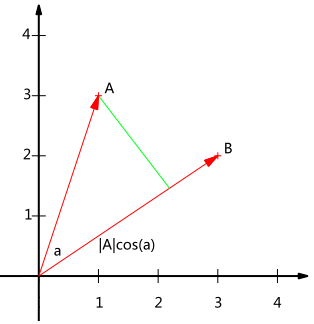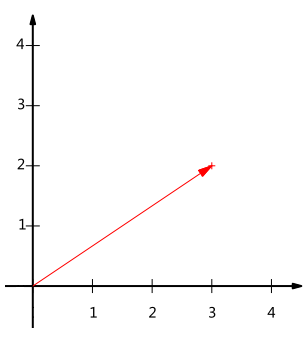图1                                                   　图2

基
本节将继续在二维空间内讨论向量。上文说过，一个二维向量可以对应二维笛卡尔直角坐标系中从原点出发的一个有向线段。例如图2所示，这个向量，在代数表示方面，我们经常用线段终点的点坐标表示向量，例如上面的向量可以表示为(3,2)，这是我们再熟悉不过的向量表示。不过我们常常忽略，只有一个(3,2)本身是不能够精确表示一个向量的。我们仔细看一下，这里的3实际表示的是向量在x轴上的投影值是3，在y轴上的投影值是2。也就是说我们其实隐式引入了一个定义：以x轴和y轴上正方向长度为1的向量为标准。那么一个向量(3,2)实际是说在x轴投影为3而y轴的投影为2。注意投影是一个矢量，所以可以为负。更正式的说，向量(x,y)实际上表示线性组合：

x(1,0)T+y(0,1)T

不难证明所有二维向量都可以表示为这样的线性组合。此处(1,0)和(0,1)叫做二维空间中的一组基，如图3。所以，要准确描述向量，首先要确定一组基，然后给出在基所在的各个直线上的投影值，就可以了。只不过我们经常省略第一步，而默认以(1,0)和(0,1)为基。我们之所以默认选择(1,0)和(0,1)为基，当然是比较方便，因为它们分别是x和y轴正方向上的单位向量，因此就使得二维平面上点坐标和向量一一对应，非常方便。但实际上任何两个线性无关的二维向量都可以成为一组基，所谓线性无关在二维平面内可以直观认为是两个不在一条直线上的向量。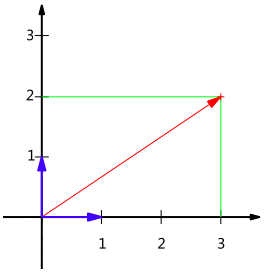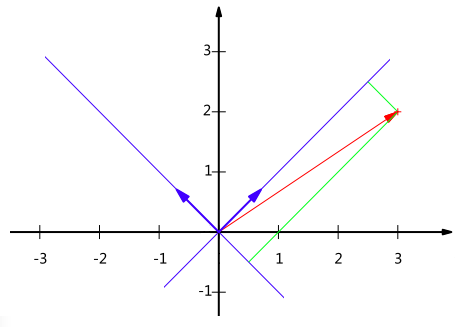图3                                                              图4

例如，(1,1)和(-1,1)也可以成为一组基。一般来说，我们希望基的模是1，因为从内积的意义可以看到，如果基的模是1，那么就可以方便的用向量点乘基而直接获得其在新基上的坐标了！实际上，对应任何一个向量我们总可以找到其同方向上模为1的向量，只要让两个分量分别除以模就好了。例如，上面的基可以变为(12√,12√)和(−12√,12√)。现在，我们想获得(3,2)在新基上的坐标，即在两个方向上的投影矢量值，那么根据内积的几何意义，我们只要分别计算(3,2)和两个基的内积，不难得到新的坐标为(52√,−12√)。图4给出了新的基以及(3,2)在新基上坐标值的示意图4所示。另外这里要注意的是，我们列举的例子中基是正交的（即内积为0，或直观说相互垂直），但可以成为一组基的唯一要求就是线性无关，非正交的基也是可以的。不过因为正交基有较好的性质，所以一般使用的基都是正交的。

基变换的矩阵表示

下面我们找一种简便的方式来表示基变换。还是拿上面的例子，想一下，将(3,2)变换为新基上的坐标，就是用(3,2)与第一个基做内积运算，作为第一个新的坐标分量，然后用(3,2)与第二个基做内积运算，作为第二个新坐标的分量。实际上，我们可以用矩阵相乘的形式简洁的表示这个变换：

(1/2√−1/2√1/2√1/2√)(32)=(5/2√−1/2√)

太漂亮了！其中矩阵的两行分别为两个基，乘以原向量，其结果刚好为新基的坐标。可以稍微推广一下，如果我们有m个二维向量，只要将二维向量按列排成一个两行m列矩阵，然后用“基矩阵”乘以这个矩阵，就得到了所有这些向量在新基下的值。例如(1,1)，(2,2)，(3,3)，想变换到刚才那组基上，则可以这样表示：

(1/2√−1/2√1/2√1/2√)(112233)=(2/2√04/2√06/2√0)

于是一组向量的基变换被干净的表示为矩阵的相乘。

一般的，如果我们有M个N维向量，想将其变换为由R个N维向量表示的新空间中，那么首先将R个基按行组成矩阵A，然后将向量按列组成矩阵B，那么两矩阵的乘积AB就是变换结果，其中AB的第m列为A中第m列变换后的结果。数学表示为：

⎛⎝⎜⎜⎜⎜p1p2⋮pR⎞⎠⎟⎟⎟⎟(a1a2⋯aM)=⎛⎝⎜⎜⎜⎜p1a1p2a1⋮pRa1p1a2p2a2⋮pRa2⋯⋯⋱⋯p1aMp2aM⋮pRaM⎞⎠⎟⎟⎟⎟

其中pi是一个行向量，表示第i个基，aj是一个列向量，表示第j个原始数据记录。

特别要注意的是，这里R可以小于N，而R决定了变换后数据的维数。也就是说，我们可以将一N维数据变换到更低维度的空间中去，变换后的维度取决于基的数量。因此这种矩阵相乘的表示也可以表示降维变换。

最后，上述分析同时给矩阵相乘找到了一种物理解释：两个矩阵相乘的意义是将右边矩阵中的每一列列向量变换到左边矩阵中每一行行向量为基所表示的空间中去。更抽象的说，一个矩阵可以表示一种线性变换。很多同学在学线性代数时对矩阵相乘的方法感到奇怪，但是如果明白了矩阵相乘的物理意义，其合理性就一目了然了。
协方差矩阵及优化目标

上述我们讨论了选择不同的基可以对同样一组数据给出不同的表示，而且如果基的数量少于向量本身的维数，则可以达到降维的效果。但是我们还没有回答一个最最关键的问题：如何选择基才是最优的。或者说，如果我们有一组N维向量，现在要将其降到K维（K小于N），那么我们应该如何选择K个基才能最大程度保留原有的信息？要完全数学化这个问题非常繁杂，这里我们用一种非形式化的直观方法来看这个问题。为了避免过于抽象的讨论，我们仍以一个具体的例子展开。假设我们的数据由五条记录组成，将它们表示成矩阵形式：

(1113234424)

其中每一列为一条数据记录，而一行为一个字段。为了后续处理方便，我们首先将每个字段内所有值都减去字段均值，其结果是将每个字段都变为均值为0（这样做的道理和好处后面会看到）。我们看上面的数据，第一个字段均值为2，第二个字段均值为3，所以变换后：

(−1−2−10002101)

我们可以看下五条数据在平面直角坐标系内的样子：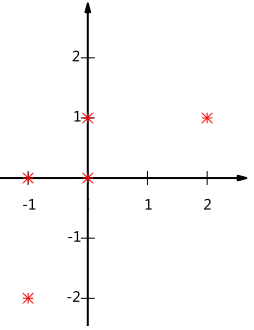现在问题来了：如果我们必须使用一维来表示这些数据，又希望尽量保留原始的信息，你要如何选择？

通过上一节对基变换的讨论我们知道，这个问题实际上是要在二维平面中选择一个方向，将所有数据都投影到这个方向所在直线上，用投影值表示原始记录。这是一个实际的二维降到一维的问题。那么如何选择这个方向（或者说基）才能尽量保留最多的原始信息呢？一种直观的看法是：希望投影后的投影值尽可能分散。以上图为例，可以看出如果向x轴投影，那么最左边的两个点会重叠在一起，中间的两个点也会重叠在一起，于是本身四个各不相同的二维点投影后只剩下两个不同的值了，这是一种严重的信息丢失，同理，如果向y轴投影最上面的两个点和分布在x轴上的两个点也会重叠。所以看来x和y轴都不是最好的投影选择。我们直观目测，如果向通过第一象限和第三象限的斜线投影，则五个点在投影后还是可以区分的。接下来，我们用数学方法表述这个问题。

方差

上文说到，我们希望投影后投影值尽可能分散，而这种分散程度，可以用数学上的方差来表述。此处，一个字段的方差可以看做是每个元素与字段均值的差的平方和的均值，即：

Var(a)=1m∑i=1m(ai−μ)2

由于上面我们已经将每个字段的均值都化为0了，因此方差可以直接用每个元素的平方和除以元素个数表示：

Var(a)=1m∑i=1ma2i

于是上面的问题被形式化表述为：寻找一个一维基，使得所有数据变换为这个基上的坐标表示后，方差值最大。

协方差

对于上面二维降成一维的问题来说，找到那个使得方差最大的方向就可以了。不过对于更高维，还有一个问题需要解决。考虑三维降到二维问题。与之前相同，首先我们希望找到一个方向使得投影后方差最大，这样就完成了第一个方向的选择，继而我们选择第二个投影方向。如果我们还是单纯只选择方差最大的方向，很明显，这个方向与第一个方向应该是“几乎重合在一起”，显然这样的维度是没有用的，因此，应该有其他约束条件。从直观上说，让两个字段尽可能表示更多的原始信息，我们是不希望它们之间存在（线性）相关性的，因为相关性意味着两个字段不是完全独立，必然存在重复表示的信息。

数学上可以用两个字段的协方差表示其相关性，由于已经让每个字段均值为0，则：

Cov(a,b)=1m∑i=1maibi

可以看到，在字段均值为0的情况下，两个字段的协方差简洁的表示为其内积除以元素数m。当协方差为0时，表示两个字段完全独立。为了让协方差为0，我们选择第二个基时只能在与第一个基正交的方向上选择。因此最终选择的两个方向一定是正交的。至此，我们得到了降维问题的优化目标：将一组N维向量降为K维（K大于0，小于N），其目标是选择K个单位（模为1）正交基，使得原始数据变换到这组基上后，各字段两两间协方差为0，而字段的方差则尽可能大（在正交的约束下，取最大的K个方差）。

协方差矩阵

上面我们导出了优化目标，但是这个目标似乎不能直接作为操作指南（或者说算法），因为它只说要什么，但根本没有说怎么做。所以我们要继续在数学上研究计算方案。我们看到，最终要达到的目的与字段内方差及字段间协方差有密切关系。因此我们希望能将两者统一表示，仔细观察发现，两者均可以表示为内积的形式，而内积又与矩阵相乘密切相关。于是我们来了灵感：假设我们只有a和b两个字段，那么我们将它们按行组成矩阵X：

X=(a1b1a2b2⋯⋯ambm)

然后我们用X乘以X的转置，并乘上系数1/m：

1mXXT=⎛⎝⎜⎜⎜⎜⎜1m∑i=1ma2i1m∑i=1maibi1m∑i=1maibi1m∑i=1mb2i⎞⎠⎟⎟⎟⎟⎟

奇迹出现了！这个矩阵对角线上的两个元素分别是两个字段的方差，而其它元素是a和b的协方差。两者被统一到了一个矩阵的。

根据矩阵相乘的运算法则，这个结论很容易被推广到一般情况：设我们有m个n维数据记录，将其按列排成n乘m的矩阵X，设C=1mXXT，则C是一个对称矩阵，其对角线分别个各个字段的方差，而第i行j列和j行i列元素相同，表示i和j两个字段的协方差。

协方差矩阵对角化

根据上述推导，我们发现要达到优化目前，等价于将协方差矩阵对角化：即除对角线外的其它元素化为0，并且在对角线上将元素按大小从上到下排列，这样我们就达到了优化目的。这样说可能还不是很明晰，我们进一步看下原矩阵与基变换后矩阵协方差矩阵的关系：设原始数据矩阵X对应的协方差矩阵为C，而P是一组基按行组成的矩阵，设Y=PX，则Y为X对P做基变换后的数据。设Y的协方差矩阵为D，我们推导一下D与C的关系：

现在事情很明白了！我们要找的P不是别的，而是能让原始协方差矩阵对角化的P。换句话说，优化目标变成了寻找一个矩阵P，满足PCPT是一个对角矩阵，并且对角元素按从大到小依次排列，那么P的前K行就是要寻找的基，用P的前K行组成的矩阵乘以X就使得X从N维降到了K维并满足上述优化条件。
现在所有焦点都聚焦在了协方差矩阵对角化问题上，由上文知道，协方差矩阵C是一个是对称矩阵，在线性代数上，实对称矩阵有一系列非常好的性质：第一、实对称矩阵不同特征值对应的特征向量必然正交；第二、设特征向量λ重数为r，则必然存在r个线性无关的特征向量对应于λ，因此可以将这r个特征向量单位正交化。由两条性质可知，一个n行n列的实对称矩阵一定可以找到n个单位正交特征向量，设这n个特征向量为e1,e2,⋯,en，我们将其按列组成矩阵：

E=(e1e2⋯en)

则对协方差矩阵C有如下结论：

ETCE=Λ=⎛⎝⎜⎜⎜⎜λ1λ2⋱λn⎞⎠⎟⎟⎟⎟

其中Λ为对角矩阵，其对角元素为各特征向量对应的特征值(可能有重复)。

因此，我们可以发现已经找到了需要的矩阵P：

P=ET

P是协方差矩阵的特征向量单位化后按行排列出的矩阵，其中每一行都是C的一个特征向量。如果设P按照Λ中特征值的从大到小，将特征向量从上到下排列，则用P的前K行组成的矩阵乘以原始数据矩阵X，就得到了我们需要的降维后的数据矩阵Y。


展开全文• ## 投影

2017-10-30 11:14:21
在查询到返回结果中，只选择必要字段，而不是选择一个文档整个字段如：一个文档有5个字段，需要显示只有3个，投影其中3个字段即可语法：参数为字段与值，值为1表示显示，值为0不显示 db.集合名称.find({},{...
投影
在查询到的返回结果中，只选择必要的字段，而不是选择一个文档的整个字段如：一个文档有5个字段，需要显示只有3个，投影其中3个字段即可语法：参数为字段与值，值为1表示显示，值为0不显示db.集合名称.find({},{字段名称:1,...})

对于需要显示的字段，设置为1即可，不设置即为不显示特殊：对于_id列默认是显示的，如果不显示需要明确设置为0例1db.stu.find({},{name:1,gender:1})

例2db.stu.find({},{_id:0,name:1,gender:1})

展开全文• 3.2 投影与反投影3.2.1 空间点正向投影反向投影（确定射线上的点的表示）3.2.2 空间直线正向投影反向投影 3.2.1 空间点 正向投影 空间点 X 通过摄像机 P 被作用到图像平面的图像点m = PX ，这种投影关系称为摄像...
• 球面投影或正视图投影是将3D点云数据表示为2D图像数据一种方式，因此从本质上讲，它还充当降维方法。球形投影方法正越来越多地用于处理点云深度学习解决方案中。应用最广泛领域是对点云中对象进行分类和分割任务...激光雷达
• 原文：http://blog.csdn.net/songzitea/article/details/18219237引言当面对数据被抽象为...首先，定义两个维数相同向量内积为：(a1,a2,⋯,an)T⋅(b1,b2,⋯,bn)T=a1b1+a2b2+⋯+anbn内积运算将两个向量映射为一...
• 给出了高斯投影和墨卡托投影正反解复变函数表达式，在此基础上推导出了这两种投影解析变换复数形式直接公式和间接公式，将其表示为含椭球第一偏心率e符号形式，可解决两种投影在不同地球参考椭球下变换...
• 直方图反向投影 1、直方图反向投影 实现步骤： 反向投影步骤： 使用到api: mixChannles(src, dst, channels); channels表示输入图像通道复制到输出图像通道， {0,0}表示输入图像第0个通道复制到输出...
• OP与以前的算法完全不同，使用新模型支持两种投影方法：基于投影的虚拟树表示，基于未过滤的投影数组表示，不仅时间效率特别高，而且特别节省了存储空间。 最后，通过Apriori，FP-Gorwth和H-Mnie的对比实验的文章...
• ## 你所不知的有趣投影方法

万次阅读 多人点赞 2016-02-04 11:48:09
高斯克吕格/Gauss Kruger、兰伯特/Lambert、墨卡托/Mercator……这些是业内人士耳熟能详 投影方法，它们各有适用场景以确保投影后某类或某几类变形最小，其最终要义就是最大程度地精确表示位置。然而，这一篇中...arcgis Map...